Chapter 21 Set Theory and General Topology

Set is the most basic concept of modern mathematics . This chapter first introduces the axiom system of set theory . However, this system has recently been proved to be incomplete , so the necessary explanation is given for the starting point taken here ( see § 1 , the last of I ) .Secondly, it introduces the main content of set theory itself-the theory of ordinal and cardinality .

Another main content of this chapter is general topology . Here we focus on several special topological spaces and point sets that are particularly important to mathematical analysis - scale space ( with a consistent structure ) , compact set, connective set, and a combination of the former two The point-to-point convergent topology, uniformly convergent topology, and compact - open topology of the transformation family are discussed . Finally, the concepts of manifolds and differential manifolds and several basic existence theorems are introduced . The knowledge of algebraic topology is not covered in this chapter (the only The exception is the notion of "simple" in § 6 ), and furthermore, the introduction of differential manifolds does not involve differential geometry (such as tangent spaces) .

§ 1 episode ( collection )

The definition of a set

1. Classical Definition of Sets

[ Set and element ] The whole of some things is called a set , and each of these things is called an element of this set ( or in this set ) .

If there is only one thing , and this thing is supposed to be denoted a , then the whole of this thing is called the set { a }, and a is the only element of { a } .

If a certain thing does not exist , the whole of such things is said to be an empty set . It is stipulated that any empty set is just the same set , denoted by φ . Nothing is an element of φ .

Each set is a thing .

[ belongs to and contains ] Suppose a is an element of set A , denoted as

a A or A a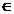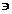" " is read as "belongs to" and " " is read as "contains" . Assuming that a is not an element of A , write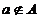or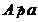" " is read as "does not belong to" and " " is read as "does not contain" .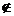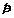[ defined note ]

{ a } and a are generally different concepts , such as { φ } has a unique element φ , but φ has no elements .

and are logically negated ( not ) of each other , in other words , suppose a is a thing and A is a set , thena A and a ANeither can be established , nor both can be established .

Assuming that A and B are both sets , if any thing belongs to A and must belong to B , and belongs to B must also belong to A , then A and B are the same set , or two sets A and B are equal , denoted as A = B. _

[ Example of set ] Suppose there are some things , all written out as a , b , c , ... , then by definition , their whole is a set , and this set can be written as { a , b , c , ... }. Element notation The order and repetition are irrelevant , such as { a , b }={ b , a }={ a , b , a }.

By definition , φ is a set , and a set is a thing , so the following things are sets :

{ φ },{{ φ }, φ },{{{{ φ }},{ φ }},{ φ }}

For another example , zero and positive integers can be defined as follows :

0 = φ

1 = { 0 } = { φ }

2 = { 0 , 1 } = { φ , { φ }}

3 = { 0 , 1 , 2 } = { φ ,{ φ },{ φ ,{ φ }}}

4 = { 0 , 1 , 2 , 3 }

…………

[ Family ] Family is a synonym for set . In some cases , such as when the elements of a set A are all sets , in order to avoid confusion , A is also called a family or a set family .

Although in modern set-theoretic models , the elements of any set are sets ( because "things" that are not sets are not considered ) , sometimes the term "family" is used to express this more clearly .

Family is also sometimes used as a quantifier . For example, all sets belonging to a set family are said to be "a family set" .

2. Russell Weird

The example above has been used to illustrate how to represent a set by enumerating elements . But when all the elements of a set cannot be enumerated , how should the set be represented ? is "the whole of all things that satisfy a certain condition" . If the sentence "a certain thing x satisfies a certain condition" is expressed as a logical formula p ( x ), then according to this customary notation , a set It can be written as { x | p ( x )} or { x : p ( x )} ( the totality of all x that makes p ( x ) true ). x , p( x ) and ( not p ( x ), that is , the negation of p ( x ) ) have one and only one true , then this notation is not a problem . But it is not the case in practice . Take the famous Russell's anomaly when Example :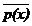Suppose . If z is a set , then z is also a thing , so z z and z z cannot both hold . Suppose z z , then z should satisfy the stated condition x x , so z z , contradicting itself . Assuming z z , then z already satisfies the stated condition x x , so z z , which contradicts itself . This is called Russell's weirdness .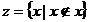According to the definition's comment , z is not a set . So the Russell weirdness is actually caused by the false assumption that " z is a set" . Apart from this formal logical reason, the Russell weirdness can be explained in more depth, but there is a fundamental The problem of is not easy to solve. Since { x|x x } is not a set, can the other { x|p ( x )} be counted as a set ?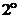To answer this question , the concept of sets must be refined further , so the axiom system is introduced below .

3.   ZFC axiom system and BNG axiom system

At present, there are two forms of the axiomatic system of set theory , one is the Zemolo - Frankel - Korean form , referred to as ZFC ; the other is the Bernes - Neumann - Gedel form , referred to as BNG . ZFC axioms are used here . system .

ZFC includes nine axioms ( three are clearly included in the definitions of the preceding sets and in the annotations of the definitions ), which are

[ Axiom of extension ] is the annotation of the definition .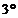[ Empty Set Axiom ] There exists a set that contains no elements .

[ Axiom of Unordered Pairs ] For anything x and y , there exists a set { x , y }, where the only elements of { x , y } are x and y .

[ Regular Axiom ] Any non-empty set A must contain an element a , and any element of A is not an element of a .

It can be known from the axiom of regularity that for any set a , a and { a } are different . This is because if a = { a }, then { a } does not conform to the axiom of regularity .

The remaining five axioms of ZFC are the axiom of substitution ( section , 2 ), the axiom of power sets of squares, the axiom of sum sets ( section , 3 ), the axiom of infinity ( § 2 , 3 ), the axiom of choice ( § 2 , 4 ). They are They are explained in detail in their respective sections . In general , these axioms specify what sets are in a more precise form . But this system of axioms cannot prove that it does not contradict itself , and it does not include all the sets necessary for set theory. are stipulated in ( § 2 , 6 ). This system therefore fails to replace the classical definition of set . The following starting points will be used later : ( i )Assume that the sets specified by these five axioms conform to the classical definition of sets and the annotations of the definitions . ( ii ) Except for sets whose elements can be enumerated in their entirety , only the sets specified by the above axioms are considered .

2.   Transformation · General notation for sets · Sets of labels

[ Ordered pair ] Assuming that x and y are things , then

< x , y> = {{ x , 1 },{ y , 2 }}

It is called an ordered pair formed by x and y , and x and y are called the first and second coordinates of < x , y > , respectively .

Ordered pairs are said for unordered pairs . It can be seen that the necessary and sufficient conditions for < x', y' > = < x, y> are : x' = x and y' = y , and the unordered pairs follow the elements one after the other The order doesn't matter .

[ Replacement axiom ] Assuming that X is a set , if for each x X as the first coordinate , there is one and only one y and x forming an ordered pair < ​​x , y > , then all the ordered pairs of the first coordinate The whole of the two-coordinate y is a set Y .Considering each < x , y> as the second coordinate of an ordered pair < x , < x , y>> , and applying the axiom of substitution again , we can see the totality of all such ordered pairs < x , y> Also a set .

[ transformation ( mapping ) image source ( original image ) image ] Assuming that X is a set , if for each x X as the first coordinate , there is one and only one y and x form an ordered pair < ​​x , y > , the whole of its second coordinate y is denoted as Y ( is a set ), then the whole of all such ordered pairs < x, y> is a set f , then f is called the transformation from X to Y ( map ), abbreviatedf is transformed ( mapped ), X is called the image source ( original ) of Y under transformation f , and Y is called the image of X under transformation f , denoted as Y = f ( X ).

In general , assuming < x , y > f , then denoted asy = f ( x )

x is called the image source of y under transformation f , and y is called the image of x under transformation f .

[ One-to-one transformation and inverse transformation ] By definition , each image source of a transformation has only one image ( uniqueness ), but an image does not necessarily have only one image source . If special, each image also has only one image source , then f is said to be a one-to-one transformation . Under a one-to-one transformation f , a transformation that changes Y to X can be obtained , which is called the inverse transformation of f . If f ( x ) = y , then ( y ) = x .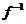[ General representation of sets and set of labels ] Suppose there is a one-to-one transformation that converts a set H into X , then X is a set , if the image of each image source h ( H ) is denoted by x h ( X ), let X beX = { x h | h H } ( 1 )Then H is called the label set of X , and each h is called the label of x h .

Conversely , a set has a label set . Because at least it can be regarded as its own label set . Therefore , the expression ( 1 ) is generally applicable . When applying this notation in the future, it is not necessary to specify H is a label set , as long as it is stipulated that the symbol written in the H position must be a label set .

3.   The set specified by the axiomatic system

[ Subset ] Assuming that A and B are both sets , and each element of B is an element of A , then B is called a subset of A , and denoted as B A or A B. " " is read as "contained in" or "Covered in", " " is read as "includes" or "covers up" .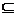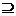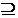For any set A , B and C have

A A ( Reflexive Law )From A B , B A , it can be deduced that A=B ( antisymmetric law )If A B , B C , then A C ( transitive law )Suppose B A but B A ( B = A does not hold ), then call B a proper subset of A , denoted by ( B A ).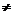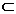Specifies that the empty set is a subset of any set .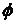[ Transformation transformation ] Suppose a transformation f transforms a set X into a subset of the upper set Y , then f is called the transformation that changes X into Y , and f for short is transformed ( mapped ). The transformation is A special case of change .

[ Division axiom and characteristic function ] Assuming that there is a transformation f that changes a set X into { 0 , 1 }, then the totality of all image sources of 1 is a subset X ' of X , and f is called the characteristic function of X' .

The division axiom is the conclusion of the substitution axiom , because if the totality of the image sources of 1 is φ , then φ is of course a subset of X , otherwise 1 has at least one image source x 0 X , making a transformation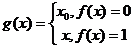Then g ( X ) = X' , so X is the set .

The corollary assumes that X is the set , and for each x X , the arguments p ( x ) and ( the negation of p ( x ) ) must hold one and only one , then { x | x X and p ( x )} is a set .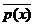[ Difference set and complement set ] Assuming that A and B are both sets , then the whole of all elements belonging to A but not B is a set ( inference by the axiom of division ), and the difference set called A and B is recorded as A \ B. _

In particular , when B is A , A \ B is called the complement of B in A.[ Axiom of Square Power Sets ] The totality of all subsets of a set A is a set , denoted as A -square power set .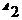One-to-one can be changed to "the set of all transformations that transform A into 2 = { 0 , 1 } ", so the latter is also a set, and this set and the A -square power set can be used as each other's label sets . In the future , they are often regarded as the same set , that is , a subset of A is confused with one of its characteristic functions .[ Axiom of Sum Set ( Union ) and Sum Set ] Assuming that { A h | h H } is a family of sets , then { x | exists an A h x } is a set , which is called the sum set ( union ) of this family of sets , recorded as .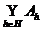When all the sets of a family are A , B , C , ... , the sum set of this family can be written as

ABC

Example      { 1 , 2 , 3 } { 0 , 2 , 4 } { 2 , 1 }={ 0 , 1 , 2 , 3 , 4 }

[ Common set ( intersection ) ] Assuming that { A h | h H } is a set family , then { x | all A h x } is a set , which is called the general set ( intersection ) of this family of sets, denoted as . The existence of a general set is the conclusion of the axiom of division .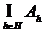When all sets of a family are A , B , C , ... , the general set of this family can be written as

ABC

Example     { 1 , 2 , 3 } { 0 , 2 , 4 } { 2 , 1 }={ 2 }

[ Direct product ( Cartesian product ) ] Assuming that A = { x h | h H }, B ={ y k | k K }, then{ < x h , y k > | x h A and y k B }is a set called the direct product of A and B , denoted A B .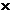Direct product existence is the conclusion of the substitution axiom and the sum set axiom . Since for any h H and k K , { < x h , y k > } is a set , by the substitution axiom , {{ < x h , y k > }| h H } is a set family , so there is a sum set . { C k | k K } is a set family , so there is a sum set , which is A B .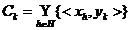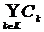Assuming that { A h | h H} is a family of sets in which each A h 1 φ , then by the axiom of choice ( §2 , a ) for each h H an x ​​h A h can be obtained , and by the axiom of substitution a set< x h | h H>= {{ x h ,h }| h H }is called an ordered group obtained by a selection transformation ( §2,4 ) .

Replace each x h A h with an x ​​' h A h , then another set is obtained by the axiom of substitution< x ' h | h H>= {{ x ' h ,h }| h H }This can also be seen as an ordered group obtained by a selection transformation .

The totality of all such ordered groups is a set, which is called the direct product of a family of sets A h ( h H ) , denoted by .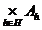When H = 2 , itis A B.[ Overlapping Sets ] Assuming that A and B are both sets , then by the definition of transformation , each transformation f that changes A into B is a subset of A B , so f . By the axiom of division , all transformations that change A into B are The whole of the transformation f { f | f and f transforms A into B } is a set , called the overlapping set that superimposes A on B , denoted A B .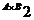Obviously , A B . On the other hand , especially when B = 2 = { 0 , 1 } , A 2 is both a square power set and a superposed set .[ Operation Law of Sets ] Assuming that A , B , and C are all sets , then

Commutative law AB = BA , AB = BA

Associativity A( BC ) = ( ΑB ) C

A( ΒC ) =( ΑB ) C

The distributive law A( BC ) = ( AB ) ( AC )

A( BC ) =( AB ) ( AC )

De Morgan 's Law _ _ _ _

C \( AB ) = ( C \ A ) ( C \ B )

C \( AB ) = ( C \ A ) ( C \ B )